# 17.18. より複雑なモデル¶

このレッスンでは、グラフィカル・モデラーにおいてより複雑なモデルで作業します。

The first model that we created in the previous chapter was a very simple one, with just one input and three algorithms. More complex models can be created, with different types of inputs and containing more steps. For this chapter we will work with a model that creates a vector layer with watersheds, based on a DEM and a threshold value. That will be very useful for calculating several vector layers corresponding to different thresholds, without having to repeat each single step each time.

This lesson does not contain instructions about how to create your model. You already know the necessary steps (we saw them in a previous lesson) and you have already seen the basic ideas about the modeler, so you should try it yourself. Spend a few minutes trying to create your model, and don't worry about making mistakes. Remember: first add the inputs and then add the algorithms that use them to create the workflow.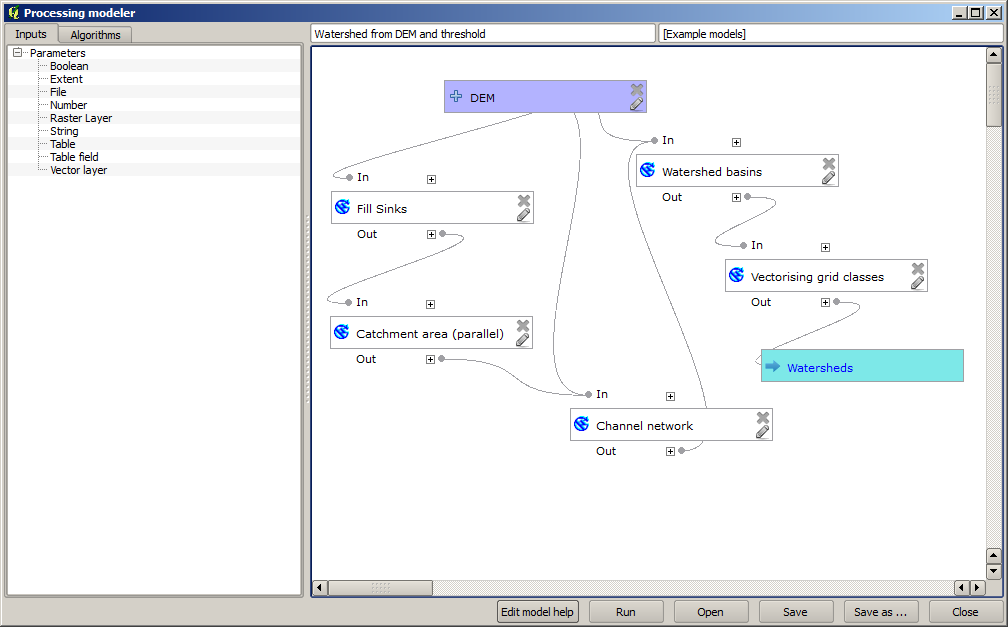This model contains all the steps needed to complete the calculation, but it just has one input: the DEM. That means that the threshold for channel definition uses a fixed value, which makes the model not as useful as it could be. That is not a problem, since we can edit the model, and that is exactly what we will do.

1. First, let's add a numerical input. That will ask the user for a numerical input that we can use when such a value is needed in any of the algorithms included in our model.

2. Click on the Number entry in the Inputs tree, and you will see the corresponding dialog.

3. Fill it with the following values.

• Parameter name: `Threshold for channel definition`

• Default value: `1,000,000`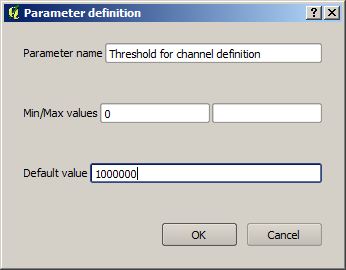今、モデルは次のようになります。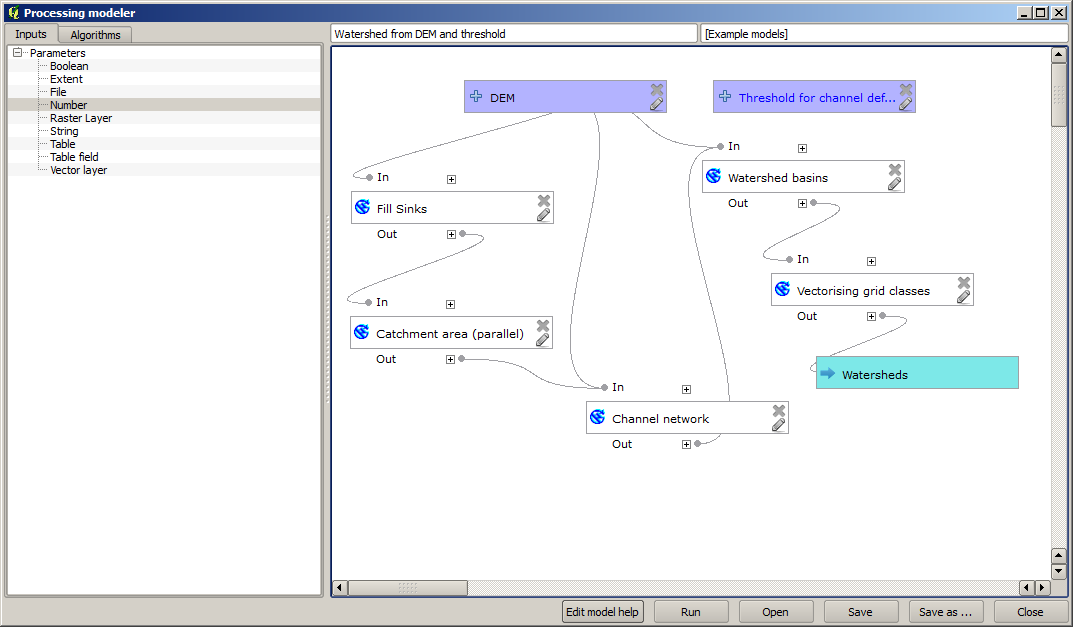The input that we have just added is not used, so the model hasn't actually changed. We have to link that input to the algorithm that uses it, in this case the Channel network one. To edit an algorithm that already exists in the modeler, just click on the pen icon on the corresponding box in the canvas.

4. Click on the Channel network algorithm and you will see something like this.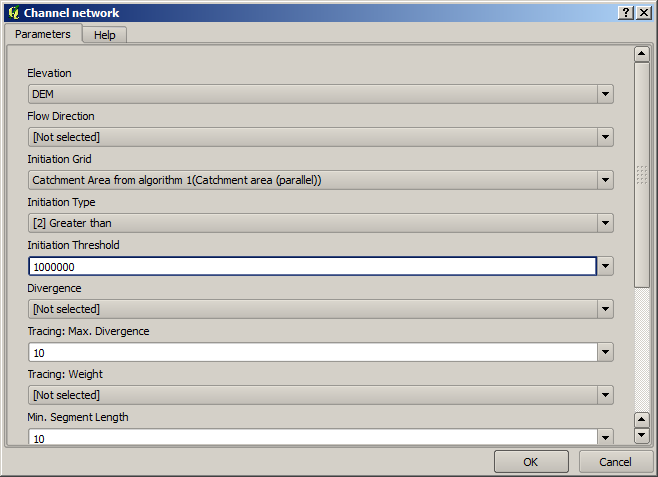The dialog is filled with the current values used by the algorithm. You can see that the Initiation threshold parameter has a fixed value of 1,000,000 (this is also the default value of the algorithm, but any other value could be put in there). However, you might notice that the parameter is not entered in a common text box, but in a drop-down menu.

5. Unfold the threshold parameter menu and you will see something like this.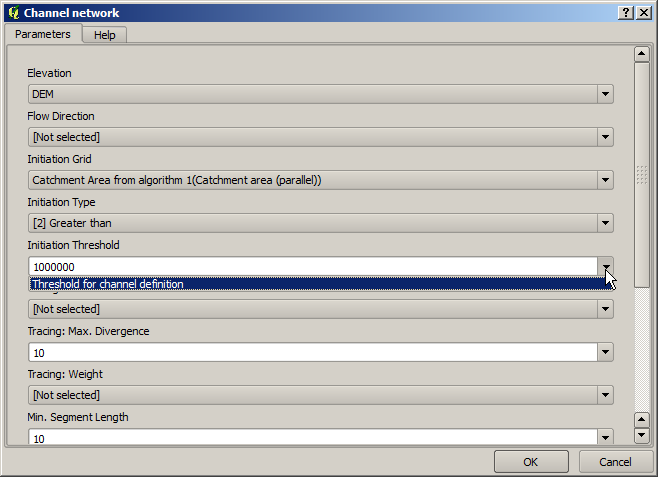追加した入力がそこにあり、それを選択できます。モデルでアルゴリズムが数値を必要とするたびに、それをハードコーディングしそれを直接入力することも、使用可能な入力と値のいずれかを使用することもできます（いくつかのアルゴリズムは単一の数値を生成することを思い出してください。これについての詳細はすぐに見るでしょう）。文字列パラメーターの場合も文字列の入力が表示され、それらのいずれかを選択することも、所望の固定値を入力することもできます。

6. Select the `Threshold for channel definition` input in the Initiation threshold parameter.

7. Click on OK to apply the changes to your model. Now the design of the model should look like this.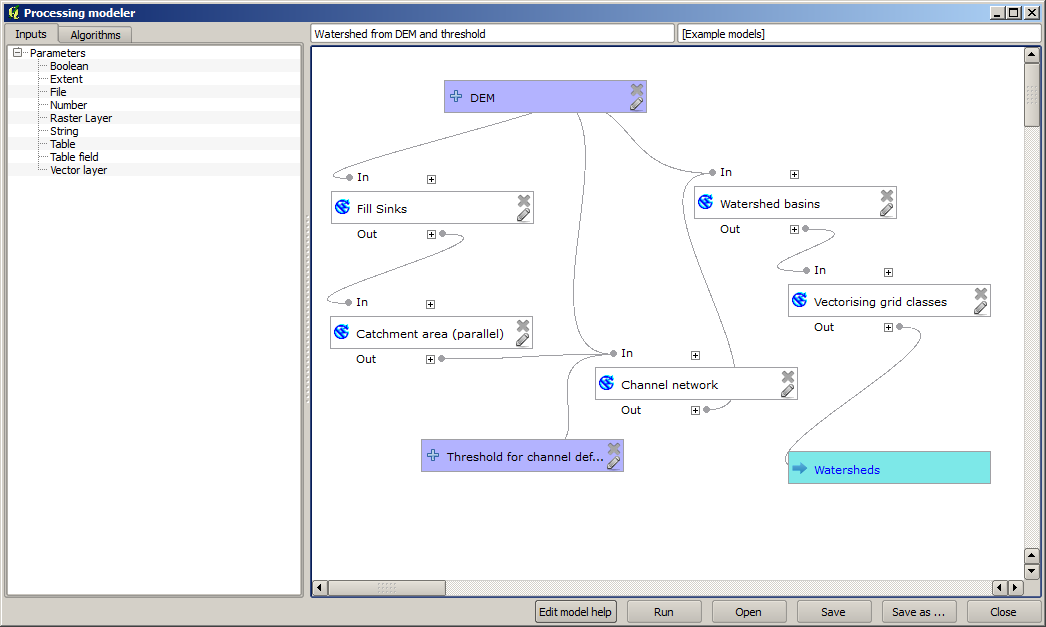8. The model is now complete. Run it using the DEM that we have used in previous lessons, and with different threshold values.

Below you have a sample of the result obtained for different values. You can compare with the result for the default value, which is the one we obtained in the hydrological analysis lesson.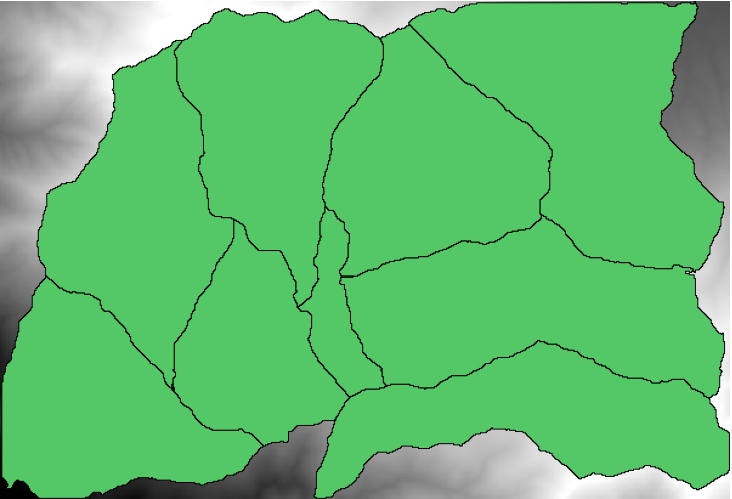図 17.2 しきい値= 100,000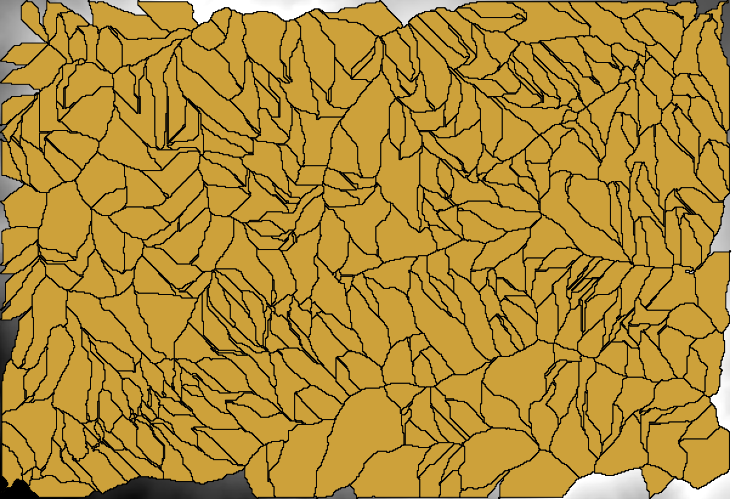図 17.3 しきい値= 1,0000,000